# Lakhmir Singh Solutions Class 9 Chemistry Chapter 3 Atoms and Molecules

The word ‘atom’ means indivisible- something which cannot be divided further. Kanad was one of the first person to propose that matter is made up of particles called ‘parmanu’. John Dalton called these particles as atoms.

All matter is made up of small particles called atoms and molecules. Different atoms and molecules have different properties, thus the properties of matter depend on properties of atoms and molecules which it is made. Atoms combine to form molecules. They are an electrically neutral group of two or more atoms chemically bond together.

A molecule is the smallest particle of a substance that has the properties of that substance. There were established many experiments that confirm the idea of atom being the smallest particle of matter. Law of chemical combination is one of the concepts came forward that comprises of three laws. Later Dalton’s atomic theory was established saying that all matter is made up of very very tiny indivisible particles called atoms. There were many assumptions and drawbacks of this theory.

This chapter deals mainly deals with the origin of atom and molecules and the various concepts of atoms, laws, and theories based on it.

## Download PDF Of Lakhmir Singh Solutions Class 9 Chemistry Chapter 3 Atoms and Molecules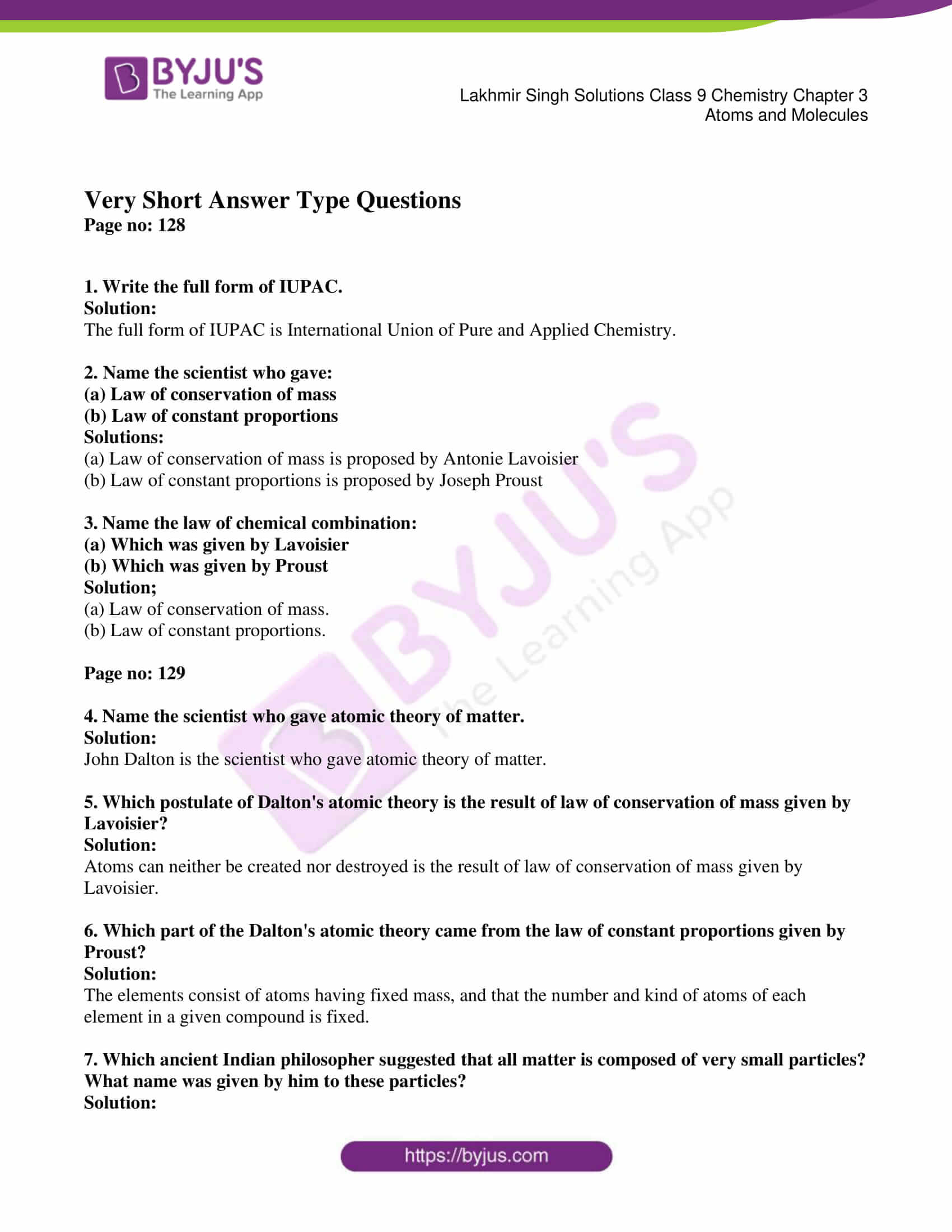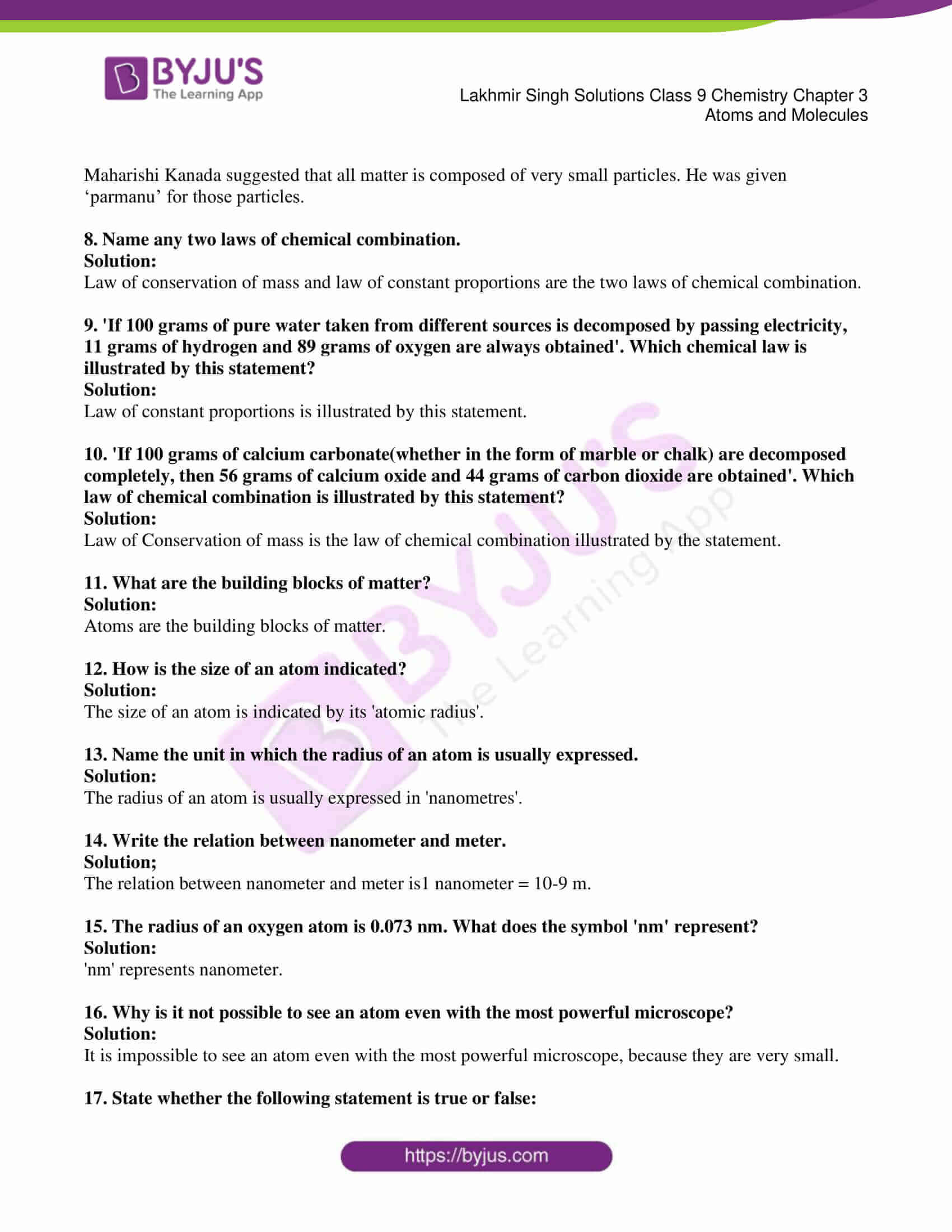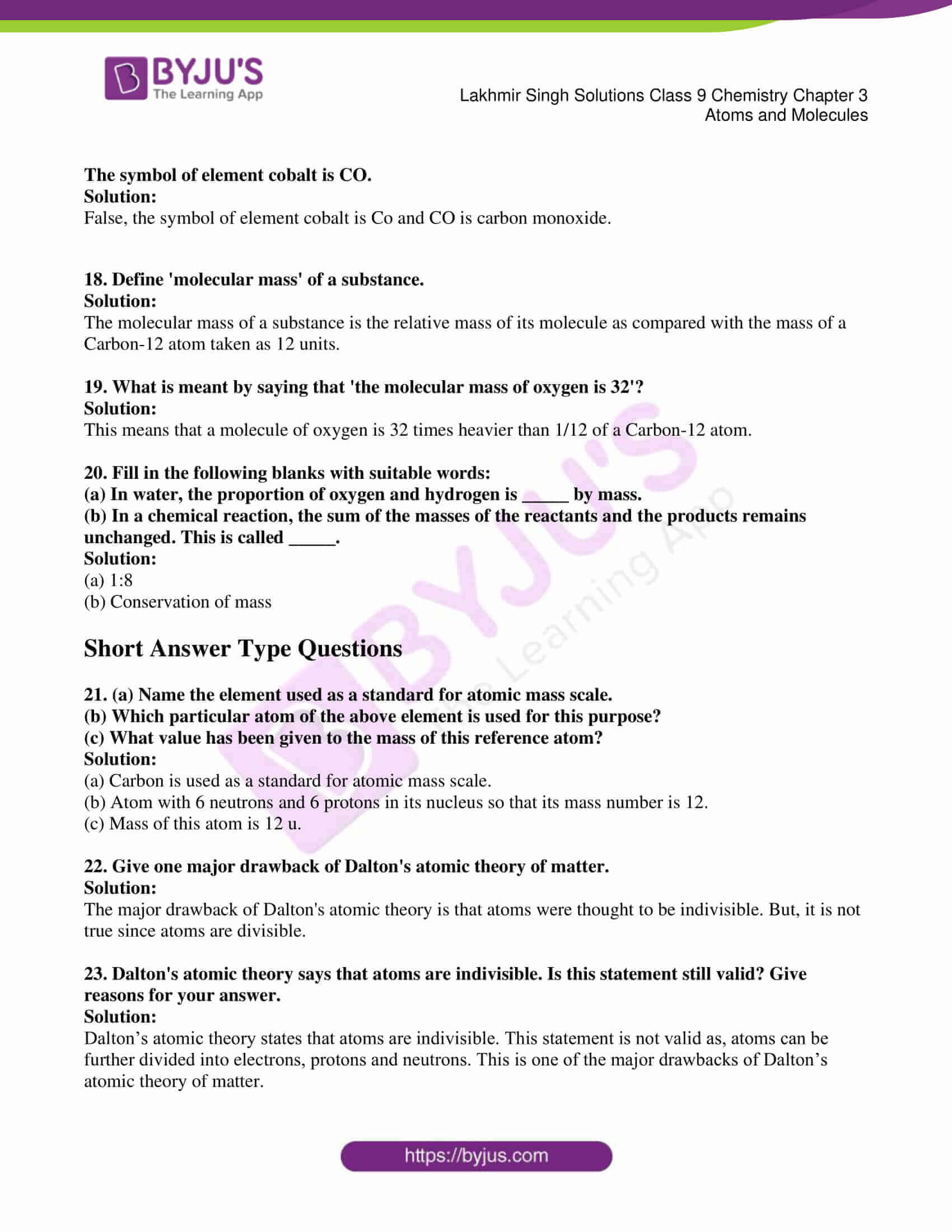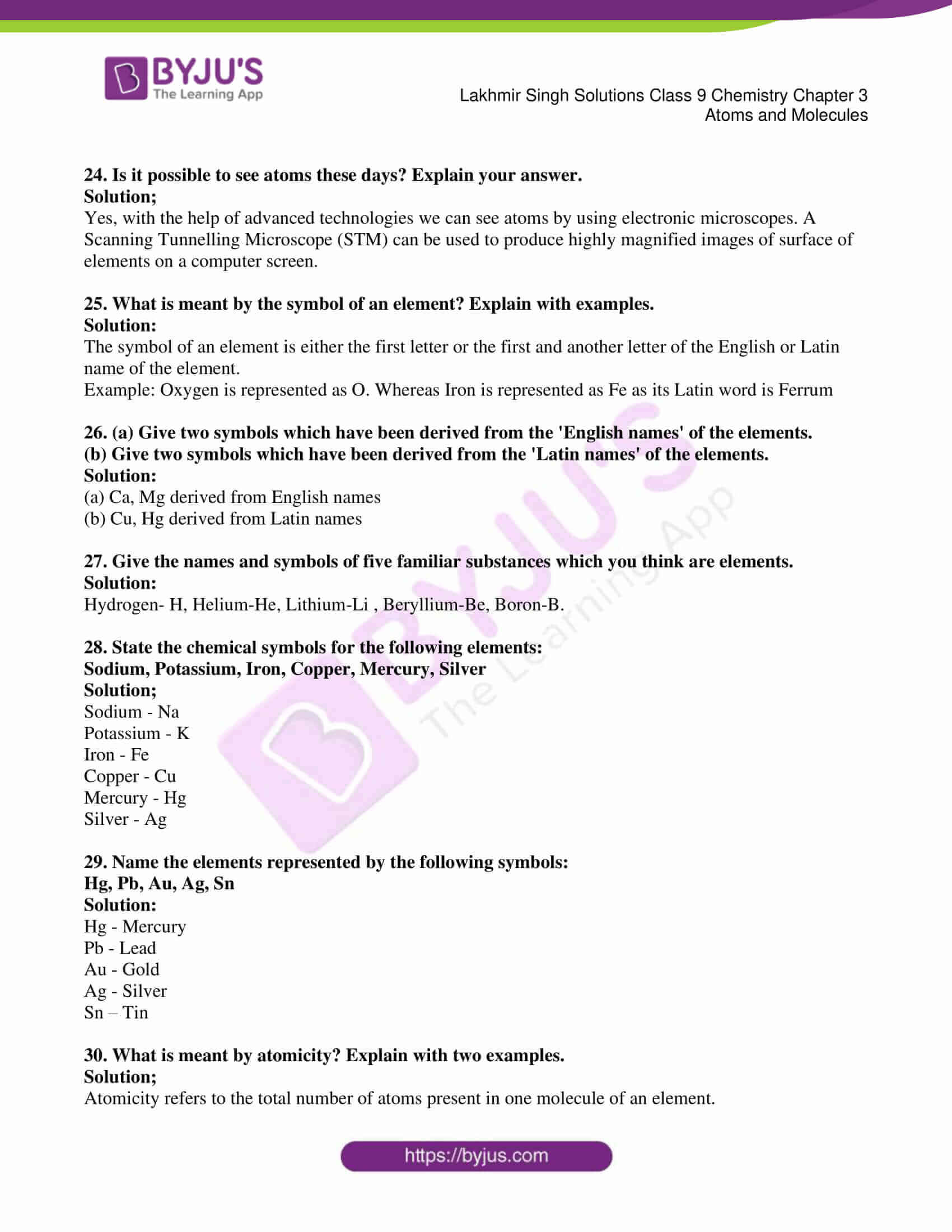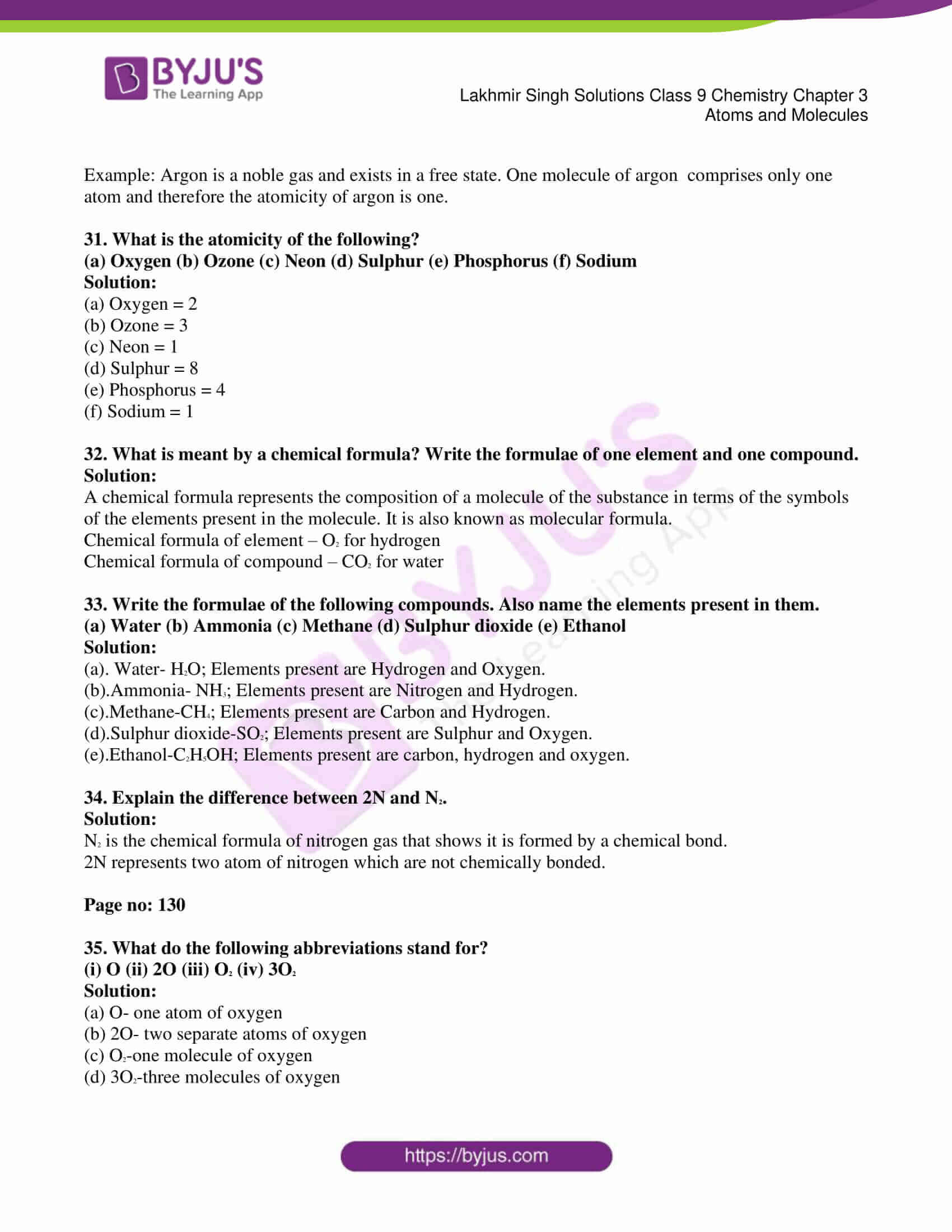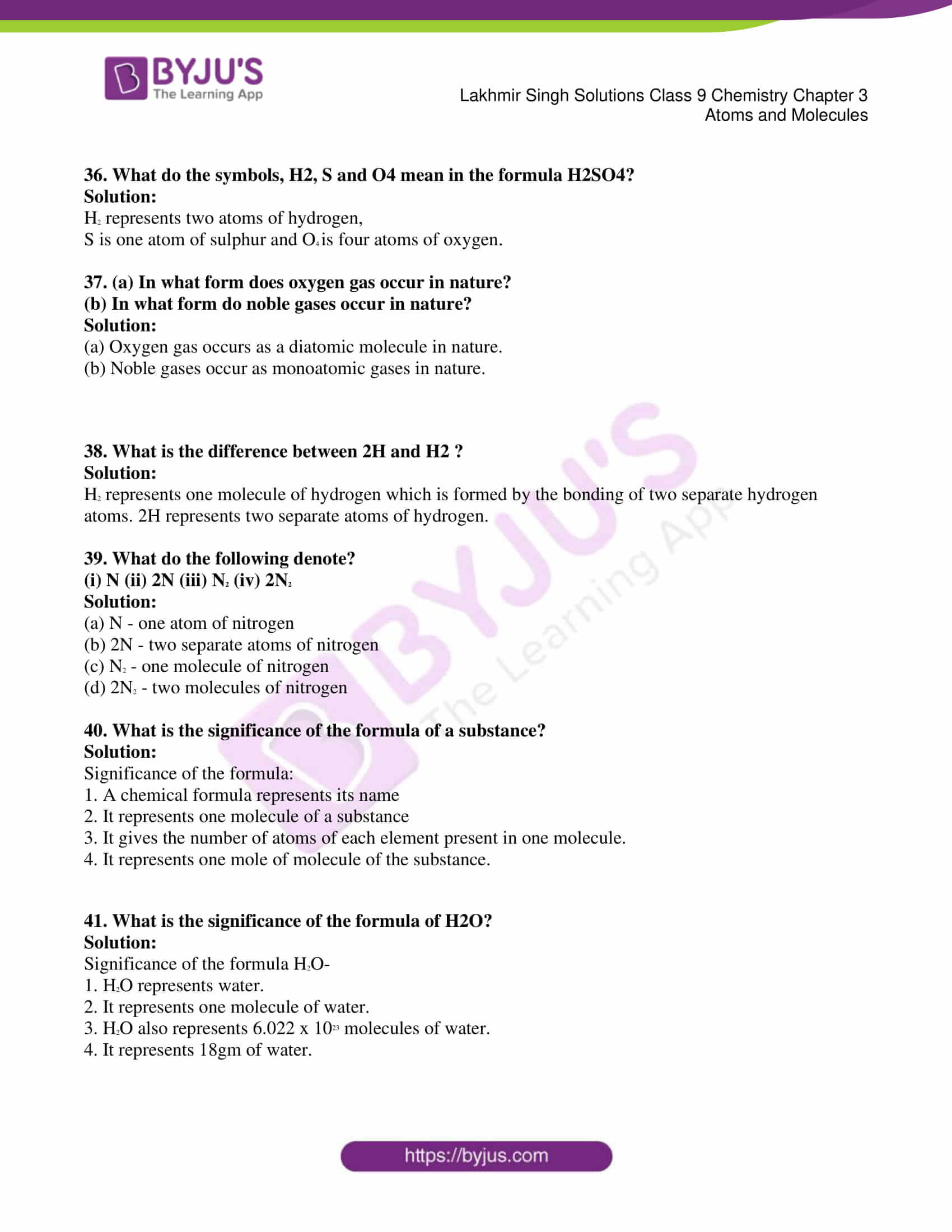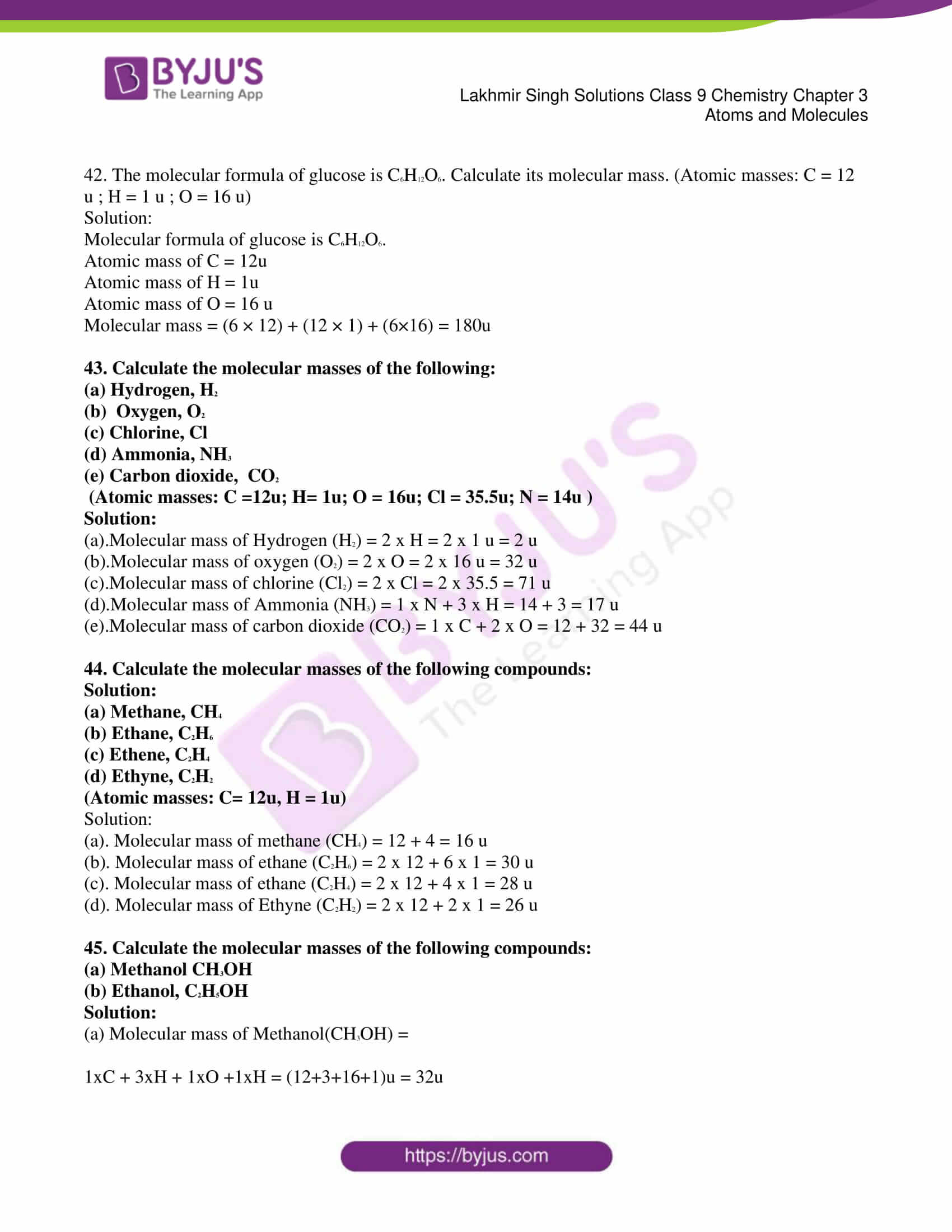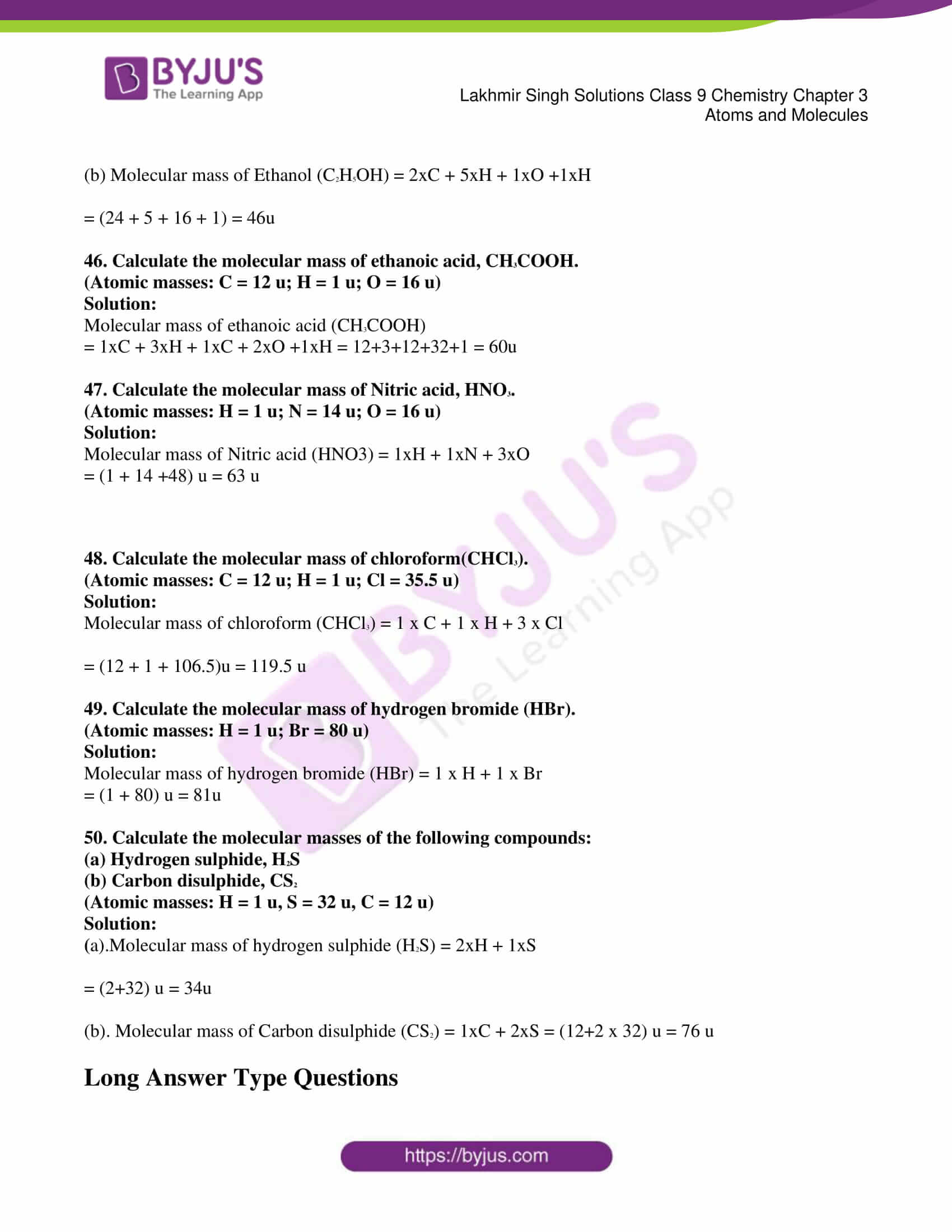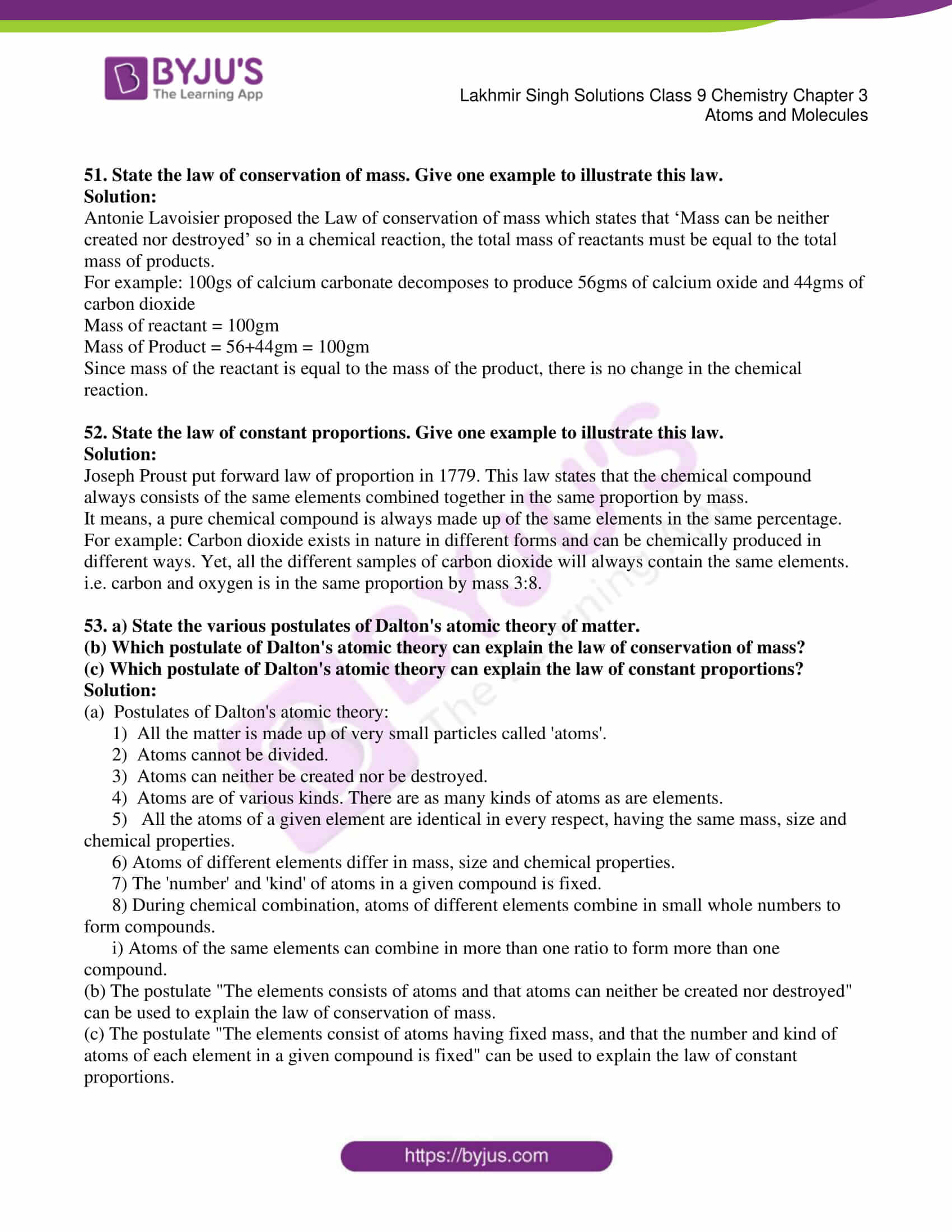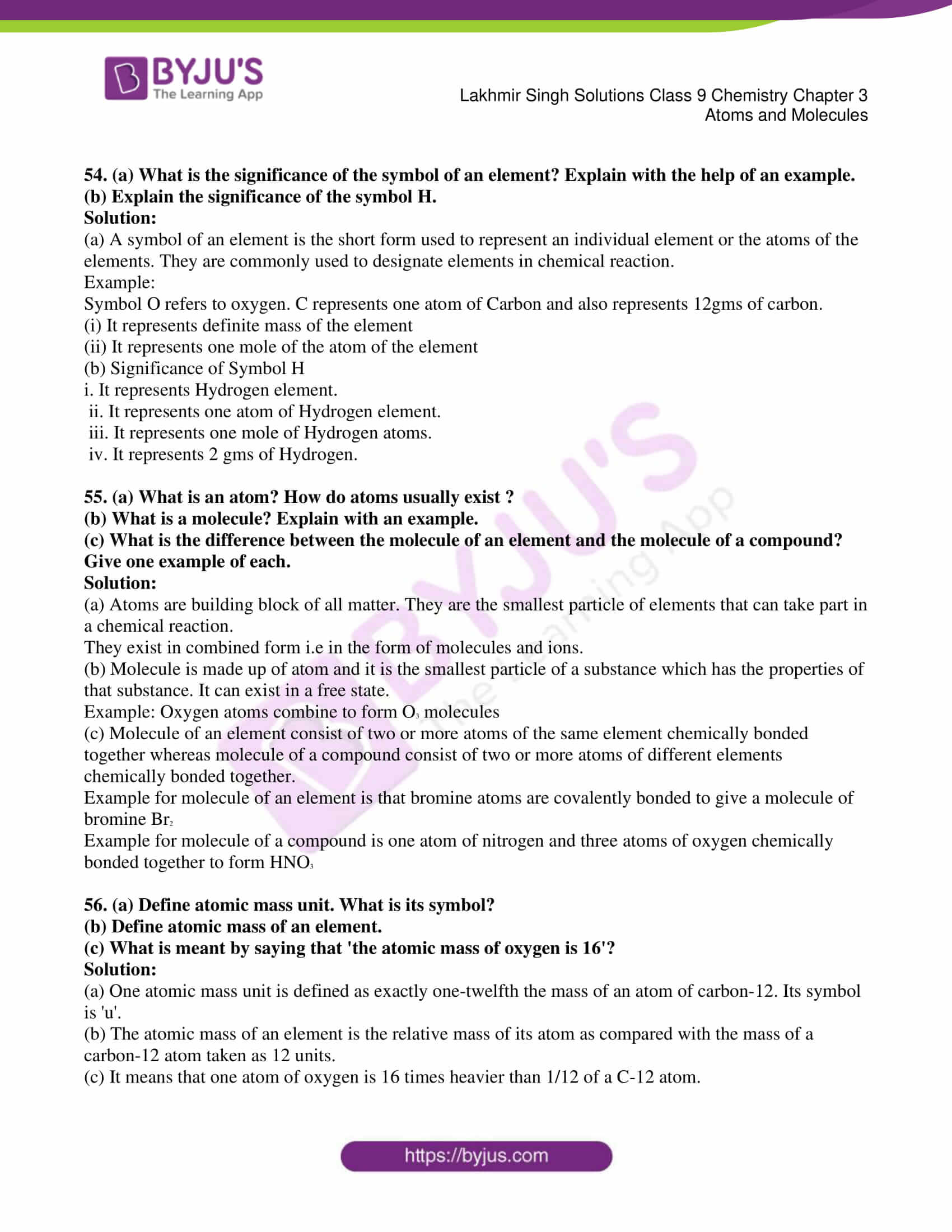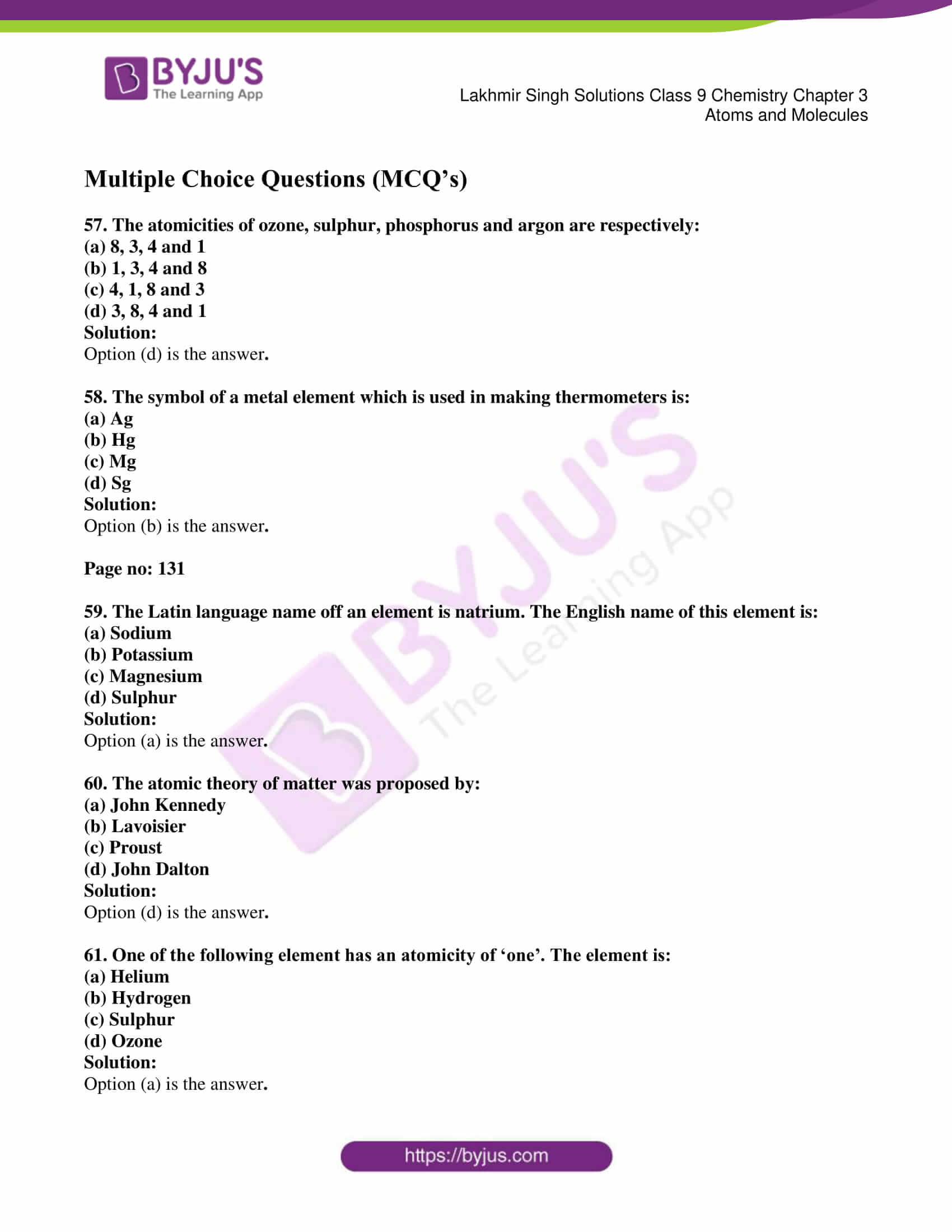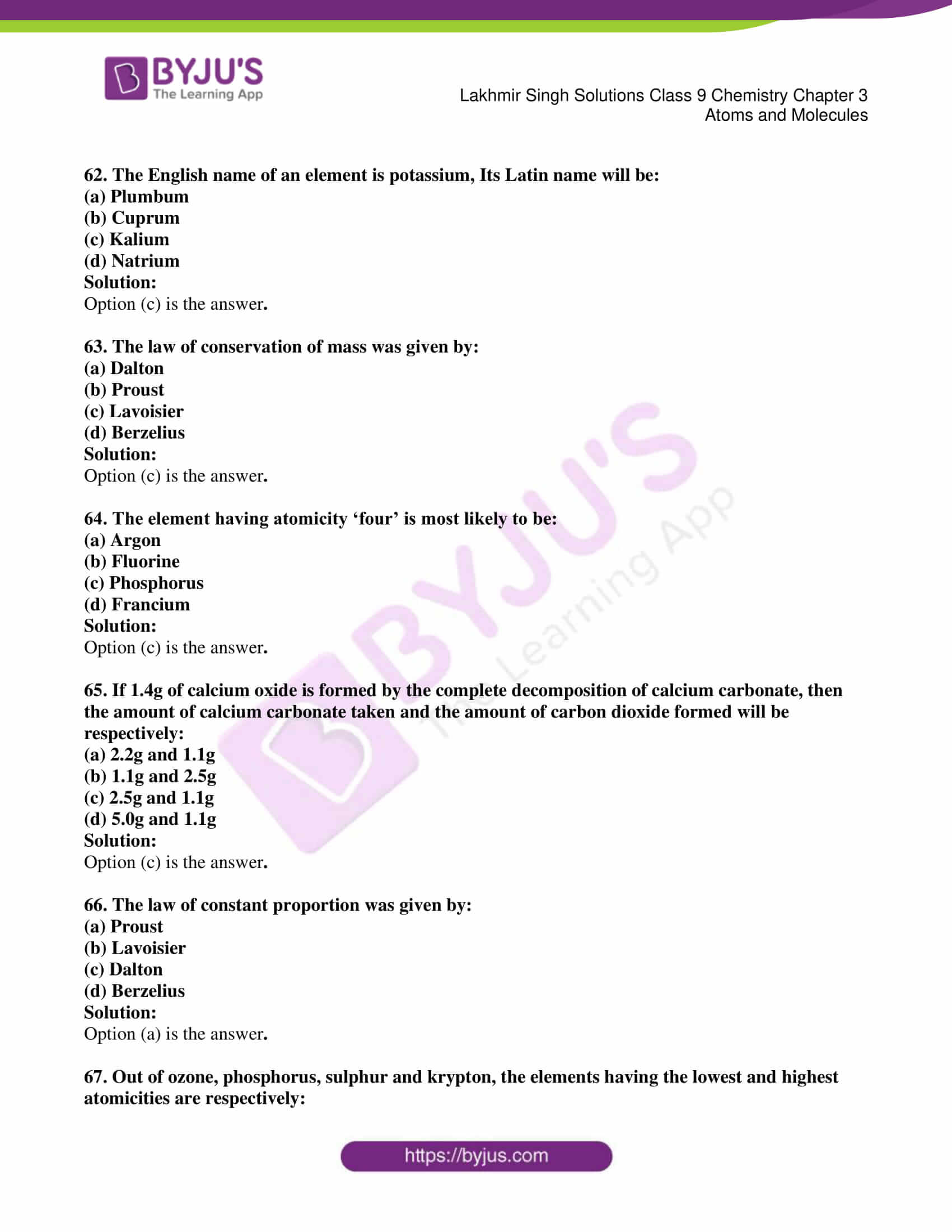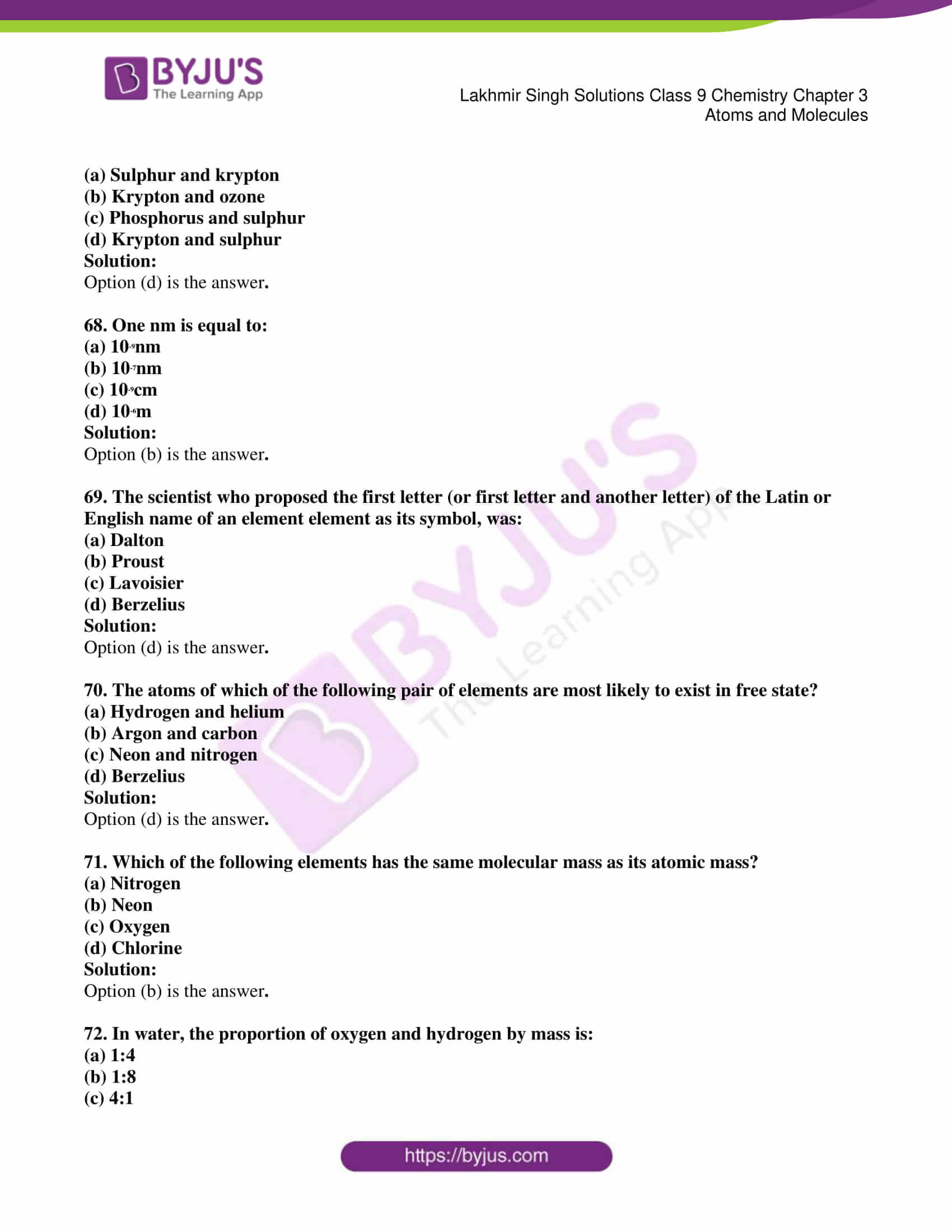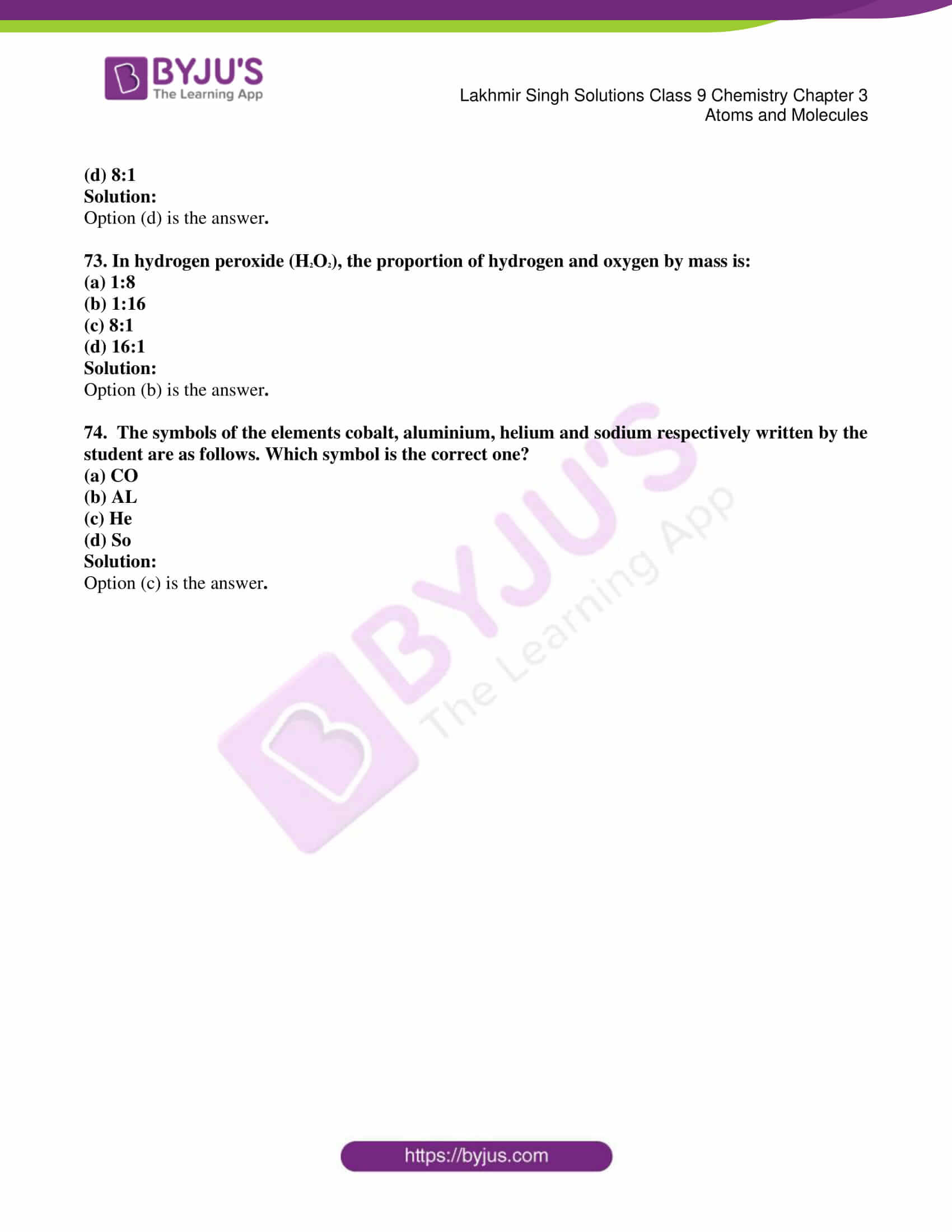### Access Lakhmir Singh Solutions Class 9 Chemistry Chapter 3 Atoms and Molecules

Page no: 128

1. Write the full form of IUPAC.

Solution:

The full form of IUPAC is International Union of Pure and Applied Chemistry.

2. Name the scientist who gave:

(a) Law of conservation of mass

(b) Law of constant proportions

Solutions:

(a) Law of conservation of mass is proposed by Antonie Lavoisier

(b) Law of constant proportions is proposed by Joseph Proust

3. Name the law of chemical combination:

(a) Which was given by Lavoisier

(b) Which was given by Proust

Solution;

(a) Law of conservation of mass.

(b) Law of constant proportions.

Page no: 129

4. Name the scientist who gave atomic theory of matter.

Solution:

John Dalton is the scientist who gave atomic theory of matter.

5. Which postulate of Dalton’s atomic theory is the result of law of conservation of mass given by Lavoisier?

Solution:

Atoms can neither be created nor destroyed is the result of law of conservation of mass given by Lavoisier.

6. Which part of the Dalton’s atomic theory came from the law of constant proportions given by Proust?

Solution:

The elements consist of atoms having fixed mass, and that the number and kind of atoms of each element in a given compound is fixed.

7. Which ancient Indian philosopher suggested that all matter is composed of very small particles? What name was given by him to these particles?

Solution:

Maharishi Kanada suggested that all matter is composed of very small particles. He was given ‘parmanu’ for those particles.

8. Name any two laws of chemical combination.

Solution:

Law of conservation of mass and law of constant proportions are the two laws of chemical combination.

9. ‘If 100 grams of pure water taken from different sources is decomposed by passing electricity, 11 grams of hydrogen and 89 grams of oxygen are always obtained’. Which chemical law is illustrated by this statement?

Solution:

Law of constant proportions is illustrated by this statement.

10. ‘If 100 grams of calcium carbonate(whether in the form of marble or chalk) are decomposed completely, then 56 grams of calcium oxide and 44 grams of carbon dioxide are obtained’. Which law of chemical combination is illustrated by this statement?

Solution:

Law of Conservation of mass is the law of chemical combination illustrated by the statement.

11. What are the building blocks of matter?

Solution:

Atoms are the building blocks of matter.

12. How is the size of an atom indicated?

Solution:

The size of an atom is indicated by its ‘atomic radius’.

13. Name the unit in which the radius of an atom is usually expressed.

Solution:

The radius of an atom is usually expressed in ‘nanometres’.

14. Write the relation between nanometer and meter.

Solution;

The relation between nanometer and meter is1 nanometer = 10-9 m.

15. The radius of an oxygen atom is 0.073 nm. What does the symbol ‘nm’ represent?

Solution:

‘nm’ represents nanometer.

16. Why is it not possible to see an atom even with the most powerful microscope?

Solution:

It is impossible to see an atom even with the most powerful microscope, because they are very small.

17. State whether the following statement is true or false:

The symbol of element cobalt is CO.

Solution:

False, the symbol of element cobalt is Co and CO is carbon monoxide.

18. Define ‘molecular mass’ of a substance.

Solution:

The molecular mass of a substance is the relative mass of its molecule as compared with the mass of a Carbon-12 atom taken as 12 units.

19. What is meant by saying that ‘the molecular mass of oxygen is 32’?

Solution:

This means that a molecule of oxygen is 32 times heavier than 1/12 of a Carbon-12 atom.

20. Fill in the following blanks with suitable words:

(a) In water, the proportion of oxygen and hydrogen is _____ by mass.

(b) In a chemical reaction, the sum of the masses of the reactants and the products remains unchanged. This is called _____.

Solution:

(a) 1:8

(b) Conservation of mass

21. (a) Name the element used as a standard for atomic mass scale.

(b) Which particular atom of the above element is used for this purpose?

(c) What value has been given to the mass of this reference atom?

Solution:

(a) Carbon is used as a standard for atomic mass scale.

(b) Atom with 6 neutrons and 6 protons in its nucleus so that its mass number is 12.

(c) Mass of this atom is 12 u.

22. Give one major drawback of Dalton’s atomic theory of matter.

Solution:

The major drawback of Dalton’s atomic theory is that atoms were thought to be indivisible. But, it is not true since atoms are divisible.

23. Dalton’s atomic theory says that atoms are indivisible. Is this statement still valid? Give reasons for your answer.

Solution:

Dalton’s atomic theory states that atoms are indivisible. This statement is not valid as, atoms can be further divided into electrons, protons and neutrons. This is one of the major drawbacks of Dalton’s atomic theory of matter.

24. Is it possible to see atoms these days? Explain your answer.

Solution;

Yes, with the help of advanced technologies we can see atoms by using electronic microscopes. A Scanning Tunnelling Microscope (STM) can be used to produce highly magnified images of surface of elements on a computer screen.

25. What is meant by the symbol of an element? Explain with examples.

Solution:

The symbol of an element is either the first letter or the first and another letter of the English or Latin name of the element.

Example: Oxygen is represented as O. Whereas Iron is represented as Fe as its Latin word is Ferrum

26. (a) Give two symbols which have been derived from the ‘English names’ of the elements.

(b) Give two symbols which have been derived from the ‘Latin names’ of the elements.

Solution:

(a) Ca, Mg derived from English names

(b) Cu, Hg derived from Latin names

27. Give the names and symbols of five familiar substances which you think are elements.

Solution:

Hydrogen- H, Helium-He, Lithium-Li , Beryllium-Be, Boron-B.

28. State the chemical symbols for the following elements:

Sodium, Potassium, Iron, Copper, Mercury, Silver

Solution;

Sodium – Na

Potassium – K

Iron – Fe

Copper – Cu

Mercury – Hg

Silver – Ag

29. Name the elements represented by the following symbols:

Hg, Pb, Au, Ag, Sn

Solution:

Hg – Mercury

Au – Gold

Ag – Silver

Sn – Tin

30. What is meant by atomicity? Explain with two examples.

Solution;

Atomicity refers to the total number of atoms present in one molecule of an element.

Example: Argon is a noble gas and exists in a free state. One molecule of argon  comprises only one atom and therefore the atomicity of argon is one.

31. What is the atomicity of the following?

(a) Oxygen (b) Ozone (c) Neon (d) Sulphur (e) Phosphorus (f) Sodium

Solution:

(a) Oxygen = 2

(b) Ozone = 3

(c) Neon = 1

(d) Sulphur = 8

(e) Phosphorus = 4

(f) Sodium = 1

32. What is meant by a chemical formula? Write the formulae of one element and one compound.

Solution:

A chemical formula represents the composition of a molecule of the substance in terms of the symbols of the elements present in the molecule. It is also known as molecular formula.

Chemical formula of element – O2 for hydrogen

Chemical formula of compound – CO2 for water

33. Write the formulae of the following compounds. Also name the elements present in them.

(a) Water (b) Ammonia (c) Methane (d) Sulphur dioxide (e) Ethanol

Solution:

(a). Water- H2O; Elements present are Hydrogen and Oxygen.

(b).Ammonia- NH3; Elements present are Nitrogen and Hydrogen.

(c).Methane-CH4; Elements present are Carbon and Hydrogen.

(d).Sulphur dioxide-SO2; Elements present are Sulphur and Oxygen.

(e).Ethanol-C2H5OH; Elements present are carbon, hydrogen and oxygen.

34. Explain the difference between 2N and N2.

Solution:

N2 is the chemical formula of nitrogen gas that shows it is formed by a chemical bond.

2N represents two atom of nitrogen which are not chemically bonded.

Page no: 130

35. What do the following abbreviations stand for?

(i) O (ii) 2O (iii) O2 (iv) 3O2

Solution:

(a) O- one atom of oxygen

(b) 2O- two separate atoms of oxygen

(c) O2-one molecule of oxygen

(d) 3O2-three molecules of oxygen

36. What do the symbols, H2, S and O4 mean in the formula H2SO4?

Solution:

H2 represents two atoms of hydrogen,

S is one atom of sulphur and O4 is four atoms of oxygen.

37. (a) In what form does oxygen gas occur in nature?

(b) In what form do noble gases occur in nature?

Solution:

(a) Oxygen gas occurs as a diatomic molecule in nature.

(b) Noble gases occur as monoatomic gases in nature.

38. What is the difference between 2H and H2 ?

Solution:

H2 represents one molecule of hydrogen which is formed by the bonding of two separate hydrogen atoms. 2H represents two separate atoms of hydrogen.

39. What do the following denote?

(i) N (ii) 2N (iii) N2 (iv) 2N2

Solution:

(a) N – one atom of nitrogen

(b) 2N – two separate atoms of nitrogen

(c) N2 – one molecule of nitrogen

(d) 2N2 – two molecules of nitrogen

40. What is the significance of the formula of a substance?

Solution:

Significance of the formula:

1. A chemical formula represents its name

2. It represents one molecule of a substance

3. It gives the number of atoms of each element present in one molecule.

4. It represents one mole of molecule of the substance.

41. What is the significance of the formula of H2O?

Solution:

Significance of the formula H2O-

1. H2O represents water.

2. It represents one molecule of water.

3. H2O also represents 6.022 x 1023 molecules of water.

4. It represents 18gm of water.

42. The molecular formula of glucose is C6H12O6. Calculate its molecular mass. (Atomic masses: C = 12 u ; H = 1 u ; O = 16 u)

Solution:

Molecular formula of glucose is C6H12O6.

Atomic mass of C = 12u

Atomic mass of H = 1u

Atomic mass of O = 16 u

Molecular mass = (6 × 12) + (12 × 1) + (6×16) = 180u

43. Calculate the molecular masses of the following:

(a) Hydrogen, H2

(b)  Oxygen, O2

(c) Chlorine, Cl

(d) Ammonia, NH3

(e) Carbon dioxide,  CO2

(Atomic masses: C =12u; H= 1u; O = 16u; Cl = 35.5u; N = 14u )

Solution:

(a).Molecular mass of Hydrogen (H2) = 2 x H = 2 x 1 u = 2 u

(b).Molecular mass of oxygen (O2) = 2 x O = 2 x 16 u = 32 u

(c).Molecular mass of chlorine (Cl2) = 2 x Cl = 2 x 35.5 = 71 u

(d).Molecular mass of Ammonia (NH3) = 1 x N + 3 x H = 14 + 3 = 17 u

(e).Molecular mass of carbon dioxide (CO2) = 1 x C + 2 x O = 12 + 32 = 44 u

44. Calculate the molecular masses of the following compounds:

Solution:

(a) Methane, CH4

(b) Ethane, C2H6

(c) Ethene, C2H4

(d) Ethyne, C2H2

(Atomic masses: C= 12u, H = 1u)

Solution:

(a). Molecular mass of methane (CH4) = 12 + 4 = 16 u

(b). Molecular mass of ethane (C2H6) = 2 x 12 + 6 x 1 = 30 u

(c). Molecular mass of ethane (C2H4) = 2 x 12 + 4 x 1 = 28 u

(d). Molecular mass of Ethyne (C2H2) = 2 x 12 + 2 x 1 = 26 u

45. Calculate the molecular masses of the following compounds:

(a) Methanol CH3OH

(b) Ethanol, C2H5OH

Solution:

(a) Molecular mass of Methanol(CH3OH) =

1xC + 3xH + 1xO +1xH = (12+3+16+1)u = 32u

(b) Molecular mass of Ethanol (C2H5OH) = 2xC + 5xH + 1xO +1xH

= (24 + 5 + 16 + 1) = 46u

46. Calculate the molecular mass of ethanoic acid, CH3COOH.

(Atomic masses: C = 12 u; H = 1 u; O = 16 u)

Solution:

Molecular mass of ethanoic acid (CH3COOH)

= 1xC + 3xH + 1xC + 2xO +1xH = 12+3+12+32+1 = 60u

47. Calculate the molecular mass of Nitric acid, HNO3.

(Atomic masses: H = 1 u; N = 14 u; O = 16 u)

Solution:

Molecular mass of Nitric acid (HNO3) = 1xH + 1xN + 3xO

= (1 + 14 +48) u = 63 u

48. Calculate the molecular mass of chloroform(CHCl3).

(Atomic masses: C = 12 u; H = 1 u; Cl = 35.5 u)

Solution:

Molecular mass of chloroform (CHCl3) = 1 x C + 1 x H + 3 x Cl

= (12 + 1 + 106.5)u = 119.5 u

49. Calculate the molecular mass of hydrogen bromide (HBr).

(Atomic masses: H = 1 u; Br = 80 u)

Solution:

Molecular mass of hydrogen bromide (HBr) = 1 x H + 1 x Br

= (1 + 80) u = 81u

50. Calculate the molecular masses of the following compounds:

(a) Hydrogen sulphide, H2S

(b) Carbon disulphide, CS2

(Atomic masses: H = 1 u, S = 32 u, C = 12 u)

Solution:

(a).Molecular mass of hydrogen sulphide (H2S) = 2xH + 1xS

= (2+32) u = 34u

(b). Molecular mass of Carbon disulphide (CS2) = 1xC + 2xS = (12+2 x 32) u = 76 u

51. State the law of conservation of mass. Give one example to illustrate this law.

Solution:

Antonie Lavoisier proposed the Law of conservation of mass which states that ‘Mass can be neither created nor destroyed’ so in a chemical reaction, the total mass of reactants must be equal to the total mass of products.

For example: 100gs of calcium carbonate decomposes to produce 56gms of calcium oxide and 44gms of carbon dioxide

Mass of reactant = 100gm

Mass of Product = 56+44gm = 100gm

Since mass of the reactant is equal to the mass of the product, there is no change in the chemical reaction.

52. State the law of constant proportions. Give one example to illustrate this law.

Solution:

Joseph Proust put forward law of proportion in 1779. This law states that the chemical compound always consists of the same elements combined together in the same proportion by mass.

It means, a pure chemical compound is always made up of the same elements in the same percentage.

For example: Carbon dioxide exists in nature in different forms and can be chemically produced in different ways. Yet, all the different samples of carbon dioxide will always contain the same elements. i.e. carbon and oxygen is in the same proportion by mass 3:8.

53. a) State the various postulates of Dalton’s atomic theory of matter.

(b) Which postulate of Dalton’s atomic theory can explain the law of conservation of mass?

(c) Which postulate of Dalton’s atomic theory can explain the law of constant proportions?

Solution:

(a)  Postulates of Dalton’s atomic theory:

1)  All the matter is made up of very small particles called ‘atoms’.

2)  Atoms cannot be divided.

3)  Atoms can neither be created nor be destroyed.

4)  Atoms are of various kinds. There are as many kinds of atoms as are elements.

5)   All the atoms of a given element are identical in every respect, having the same mass, size and chemical properties.

6) Atoms of different elements differ in mass, size and chemical properties.

7) The ‘number’ and ‘kind’ of atoms in a given compound is fixed.

8) During chemical combination, atoms of different elements combine in small whole numbers to form compounds.

i) Atoms of the same elements can combine in more than one ratio to form more than one compound.

(b) The postulate “The elements consists of atoms and that atoms can neither be created nor destroyed” can be used to explain the law of conservation of mass.

(c) The postulate “The elements consist of atoms having fixed mass, and that the number and kind of atoms of each element in a given compound is fixed” can be used to explain the law of constant proportions.

54. (a) What is the significance of the symbol of an element? Explain with the help of an example.

(b) Explain the significance of the symbol H.

Solution:

(a) A symbol of an element is the short form used to represent an individual element or the atoms of the elements. They are commonly used to designate elements in chemical reaction.

Example:

Symbol O refers to oxygen. C represents one atom of Carbon and also represents 12gms of carbon.

(i) It represents definite mass of the element

(ii) It represents one mole of the atom of the element

(b) Significance of Symbol H

i. It represents Hydrogen element.

ii. It represents one atom of Hydrogen element.

iii. It represents one mole of Hydrogen atoms.

iv. It represents 2 gms of Hydrogen.

55. (a) What is an atom? How do atoms usually exist ?

(b) What is a molecule? Explain with an example.

(c) What is the difference between the molecule of an element and the molecule of a compound? Give one example of each.

Solution:

(a) Atoms are building block of all matter. They are the smallest particle of elements that can take part in a chemical reaction.

They exist in combined form i.e in the form of molecules and ions.

(b) Molecule is made up of atom and it is the smallest particle of a substance which has the properties of that substance. It can exist in a free state.

Example: Oxygen atoms combine to form O3 molecules

(c) Molecule of an element consist of two or more atoms of the same element chemically bonded together whereas molecule of a compound consist of two or more atoms of different elements chemically bonded together.

Example for molecule of an element is that bromine atoms are covalently bonded to give a molecule of bromine Br2

Example for molecule of a compound is one atom of nitrogen and three atoms of oxygen chemically bonded together to form HNO3

56. (a) Define atomic mass unit. What is its symbol?

(b) Define atomic mass of an element.

(c) What is meant by saying that ‘the atomic mass of oxygen is 16’?

Solution:

(a) One atomic mass unit is defined as exactly one-twelfth the mass of an atom of carbon-12. Its symbol is ‘u’.

(b) The atomic mass of an element is the relative mass of its atom as compared with the mass of a carbon-12 atom taken as 12 units.

(c) It means that one atom of oxygen is 16 times heavier than 1/12 of a C-12 atom.

Multiple Choice Questions (MCQ’s)

57. The atomicities of ozone, sulphur, phosphorus and argon are respectively:

(a) 8, 3, 4 and 1

(b) 1, 3, 4 and 8

(c) 4, 1, 8 and 3

(d) 3, 8, 4 and 1

Solution:

58. The symbol of a metal element which is used in making thermometers is:

(a) Ag

(b) Hg

(c) Mg

(d) Sg

Solution:

Page no: 131

59. The Latin language name off an element is natrium. The English name of this element is:

(a) Sodium

(b) Potassium

(c) Magnesium

(d) Sulphur

Solution:

60. The atomic theory of matter was proposed by:

(a) John Kennedy

(b) Lavoisier

(c) Proust

(d) John Dalton

Solution:

61. One of the following element has an atomicity of ‘one’. The element is:

(a) Helium

(b) Hydrogen

(c) Sulphur

(d) Ozone

Solution:

62. The English name of an element is potassium, Its Latin name will be:

(a) Plumbum

(b) Cuprum

(c) Kalium

(d) Natrium

Solution:

63. The law of conservation of mass was given by:

(a) Dalton

(b) Proust

(c) Lavoisier

(d) Berzelius

Solution:

64. The element having atomicity ‘four’ is most likely to be:

(a) Argon

(b) Fluorine

(c) Phosphorus

(d) Francium

Solution:

65. If 1.4g of calcium oxide is formed by the complete decomposition of calcium carbonate, then the amount of calcium carbonate taken and the amount of carbon dioxide formed will be respectively:

(a) 2.2g and 1.1g

(b) 1.1g and 2.5g

(c) 2.5g and 1.1g

(d) 5.0g and 1.1g

Solution:

66. The law of constant proportion was given by:

(a) Proust

(b) Lavoisier

(c) Dalton

(d) Berzelius

Solution:

67. Out of ozone, phosphorus, sulphur and krypton, the elements having the lowest and highest atomicities are respectively:

(a) Sulphur and krypton

(b) Krypton and ozone

(c) Phosphorus and sulphur

(d) Krypton and sulphur

Solution:

68. One nm is equal to:

(a) 10-9nm

(b) 10-7nm

(c) 10-9cm

(d) 10-6m

Solution:

69. The scientist who proposed the first letter (or first letter and another letter) of the Latin or English name of an element element as its symbol, was:

(a) Dalton

(b) Proust

(c) Lavoisier

(d) Berzelius

Solution:

70. The atoms of which of the following pair of elements are most likely to exist in free state?

(a) Hydrogen and helium

(b) Argon and carbon

(c) Neon and nitrogen

(d) Berzelius

Solution:

71. Which of the following elements has the same molecular mass as its atomic mass?

(a) Nitrogen

(b) Neon

(c) Oxygen

(d) Chlorine

Solution:

72. In water, the proportion of oxygen and hydrogen by mass is:

(a) 1:4

(b) 1:8

(c) 4:1

(d) 8:1

Solution:

73. In hydrogen peroxide (H2O2), the proportion of hydrogen and oxygen by mass is:

(a) 1:8

(b) 1:16

(c) 8:1

(d) 16:1

Solution:

74.  The symbols of the elements cobalt, aluminium, helium and sodium respectively written by the student are as follows. Which symbol is the correct one?

(a) CO

(b) AL

(c) He

(d) So

Solution:

Also, visit Lakhmir Singh Solutions for Class 9 Chemistry to get complete solutions for all chapters.